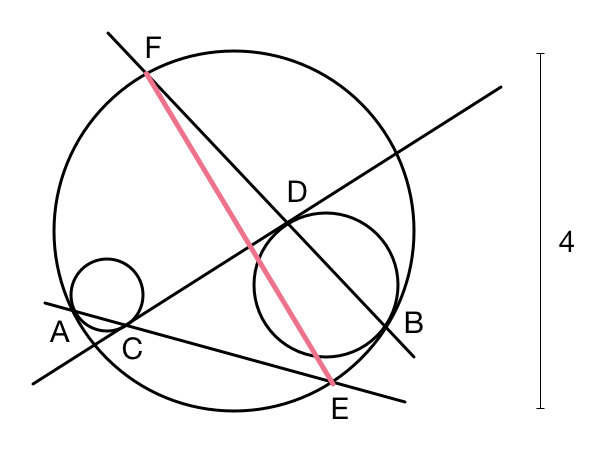# Too Ugly?

Geometry Level 1

In a large circle of diameter 4, we draw two circles that are each internally tangent at $A$ and $B.$ We then draw the common interior tangent $CD.$ If $AC$ intersects the large circle at $E$ and $BD$ intersects the large circle at $F,$ what is the distance $EF?$×

Problem Loading...

Note Loading...

Set Loading...